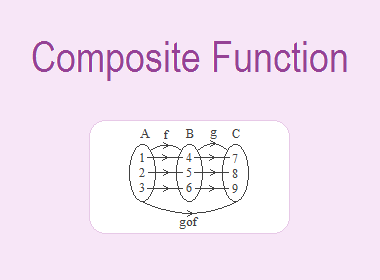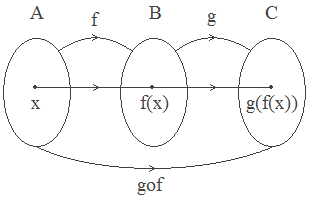# Composite FunctionLet A = {2, 3, 4}, B = {6, 9, 12} and C = {a, b, c}. If f : A → B and g : B → C are defined by f = {(2, 6), (3, 9), (4, 12)} and g = {(6, a), (9, b), (12, c)},f is the function from A to B such that

2 A, f(2) = 6 B

3 A, f(3) = 9 B

4 A, f(4) = 12 B

Range of f = {6, 9, 12} = domain of g.

Again, g is the function from B to C such that

6 B, g(6) = a C

9 B, g(9) = b C

12 B, g(12) = c C********************

********************

Now, a function defined from A to C such that

2 A, a = g(6) = g(f(2)) C

3 A, b = g(9) = g(f(3)) C

4 A, c = g(12) = g(f(4)) C

Then this new function defined from set A to set C is said to be a composite function of f and g and is denoted by gof or simply gf. Hence, we have (gof)(2) = a, (gof)(3) = b, (gof)(4) = c.

## Definition of Composite Function:

Let f : A → B and g : B → C be two functions. Then a new function defined from A to C such that every element of A corresponds with a unique element of C is known as the composite function of f and g. It is denoted by gof or simply gf.### Worked Out Examples:

Example 1: If f = {(2, 4), (6, 10), (8, 2)} and g = {(4, 6), (10, 2), (2, 6)}, then show that the function fog and gof in arrow diagram and find it in ordered pair form.

Solution:

Here,

For fog

fog(4) = f(g(4)) = f(6) = 10

fog(10) = f(g(10)) = f(2) = 4

fog(2) = f(g(2)) = f(6) = 10

Hence, fog = {(4, 10), (10, 4), (2, 10)}

For gof,

gof(2) = g(f(2)) = g(4) = 6

gof(6) = g(f(6)) = g(10) = 2

gof(8) = g(f(8)) = g(2) = 6

Hence, gof = {(2, 6), (6, 2), (8, 6)}

Example 2: If f : R → R and g : R → R be the two functions defined by f(x) = 3x + 7 and g(x) = 2(x – 8), find gof(x) and fog(x) and test whether gof(x) = fog(x) or not.

Solution:

Here,

f(x) = 3x + 7 and g(x) = 2(x – 8).

Now,

gof(x)         = g(f(x))

= g(3x + 7)

= 2{(3x + 7) – 8}

= 2 (3x – 1)

= 6x – 2

gof(x) = 6x – 2

And,

fog(x)         = f(g(x))

= f(2(x – 8))

= f(2x – 16)

= 3(2x – 16) + 7

= 6x – 48 + 7

= 6x – 41

fog(x) = 6x – 41

Hence, gof(x) ≠ fog(x)

Example 3: If f(x) = 2x – 1, g(x) = 3x and h(x) = x + 3, find

a. fogoh(x)

b. gofoh(2)

Solution:

Here,

We have, f(x) = 2x – 1, g(x) = 3x and h(x) = x + 3

a. fogoh(x) = f(goh(x))

= f(g(h(x)))

= f(g(x + 3))

= f(3(x + 3))

= f(3x + 9)

= 2(3x + 9) – 1

= 6x + 18 – 1

= 6x + 17

b. gofoh(2) = g(foh(2))

= g(f(h(2)))

= g(f(2 + 3))

= g(f(5))

= g(2 × 5 – 1)

= g(9)

= 3 × 9

= 27